# 实现一个二叉搜索树（JavaScript 版）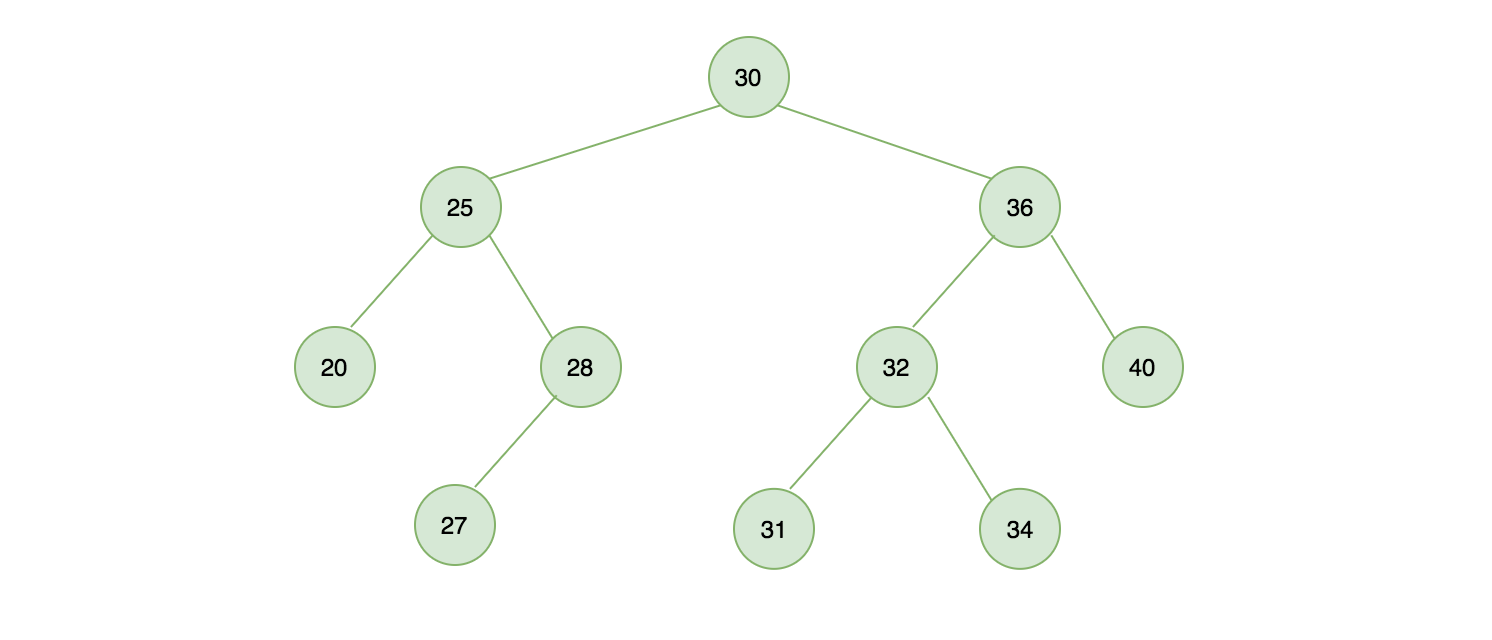## 二叉搜索树实现大纲

• constructor()：构造函数，初始化一个二叉搜索树
• insert(value)：二叉树中查找一个节点，如果存在返回 true 否则返回 false
• preOrderTraverse(cb)：先序遍历或称前序遍历
• inOrderTraverse(cb)：中序遍历
• postOrderTraverse(cb)：后序遍历
• minNodeValue()：最小节点值
• maxNodeValue()：最大节点值
• removeNode(value)：移除节点
• destory()：销毁节点

## 初始化一个二叉搜索树

```class BST {
constructor () {
this.root = null; // 初始化根节点
this.count = 0; // 记录二叉搜索的节点数量

/**
* 实例化一个 node 节点，在 insert 方法中你会看到
*/
this.Node = function(value) {
return {
value, // 节点值
count: 1, // 节点数量，允许节点重复
left: null, // 左侧子节点
right: null, // 右侧子节点
}
}
}
```

## 二叉搜索树插入节点

```/**
* 二叉搜索树插入元素
* @param { Number } value
*/
insert(value) {
this.root = this[INSERT_RECUSIVE](this.root, value);
}
```

INSERT_RECUSIVE 使用 Symbol 进行声明

```const INSERT_RECUSIVE = Symbol('BST#recursiveInsert');
```

```/**
* 递归插入
* 插入过程和链表类似，建议先学习链表会更容易理解
* @param { Object } node
* @param { Number } value
*/
[INSERT_RECUSIVE](node, value) {
// {1} 如果当前节点为空，创建一个新节点（递归到底）
if (node === null) {
this.count++; // 节点数加 1
return new this.Node(value);
}

// {2} 节点数不变，说明要更新的值等于二叉树中的某个节点值
if (value === node.value) {
node.count++; // 节点数加 1
} else if (value < node.value) { // {3} 新插入子节点在二叉树左边，继续递归插入
node.left = this[INSERT_RECUSIVE](node.left, value);
} else if (value > node.value) { // {4} 新插入子节点在二叉树右边，继续递归插入
node.right = this[INSERT_RECUSIVE](node.right, value);
}

return node;
}
```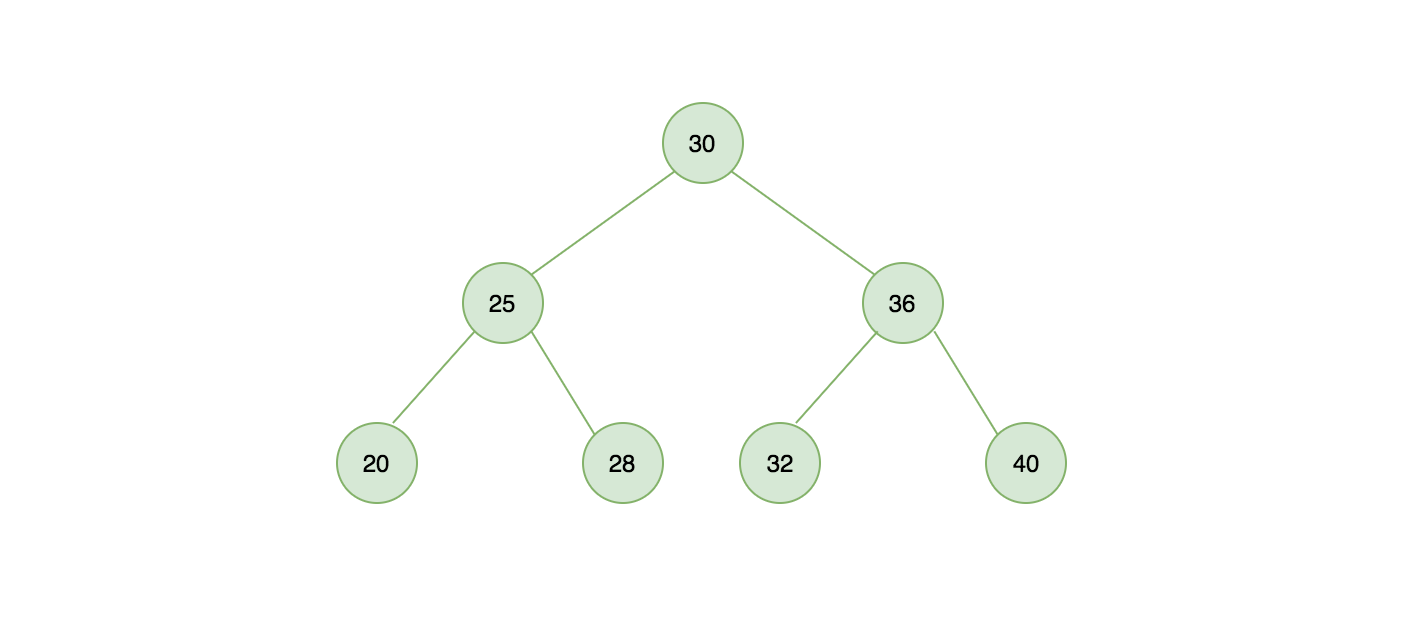• 第一次执行 bST.insert(30) 树是空的，代码行 {1} 将会被执行调用 new this.Node(value) 插入一个新节点。
• 第二次执行 bST.insert(25) 树不是空的，25 比 30 小（value < node.value），代码行 {3} 将会被执行，在树的左侧递归插入并调用 INSERT_RECUSIVE 方法传入 node.left，第二次递归时由于 node.left 已经为 null，所以插入新节点
• 第三次执行 bST.insert(36) 同理，执行顺序为 {4} -> 递归 {1}
```const bST = new BST();

bST.insert(30);
bST.insert(25);
bST.insert(36);
bST.insert(20);
bST.insert(28);
bST.insert(32);
bST.insert(40);

console.dir(bST, { depth: 4 })
```

## 二叉搜索树查找节点

JavaScript 中我们可以通过 hasOwnProperty 来检测指定 key 在对象是否存在，现在我们在二叉搜索中实现一个类似的方法，传入一个值 value 判断是否在二叉搜索树中存在

```/**
* 二叉树中搜索节点
* @param { Number } value
* @return { Boolean } [true|false]
*/
search(value) {
return this[SEARCH_RECUSIVE](this.root, value);
}
```

• 行 {1} 先判断传入的 node 是否为 null，如果为 null 就表示查找失败，返回 false。
• 行 {2} 说明已经找到了节点，返回 true。
• 行 {3} 表示要找的节点，比当前节点小，在左侧节点继续查找。
• 行 {4} 表示要找的节点，比当前节点大，在右侧节点继续查找。
```/**
* 递归搜索
* @param { Object } node
* @param { Number } value
*/
[SEARCH_RECUSIVE](node, value) {
if (node === null) { // {1} 节点为 null
return false;
} else if (value === node.value) { // {2} 找到节点
return true;
} else if (value < node.value) { // {3} 从左侧节点搜索
return this[SEARCH_RECUSIVE](node.left, value);
} else { // {4} 从右侧节点搜索
return this[SEARCH_RECUSIVE](node.right, value);
}
}
```

```bST.search(20); // true
bST.search(10); // false
```

## 二叉搜索树遍历

### 先序遍历

```/**
* 先序遍历（前序遍历）
* @param { Function } cb
*/
preOrderTraverse(cb) {
return this[PRE_ORDER_TRAVERSE_RECUSIVE](this.root, cb);
}
```

• 行 {1} 先访问节点本身（从树的顶端开始）
• 行 {2} 访问左侧节点
• 行 {3} 访问右侧节点
```/**
* 先序遍历递归调用
* @param { Object } node
* @param { Function } cb
*/
[PRE_ORDER_TRAVERSE_RECUSIVE](node, cb) {
if (node !== null) {
cb(node.value); // {1} 先访问节点本身（从树的顶端开始）
this[PRE_ORDER_TRAVERSE_RECUSIVE](node.left, cb); // {2} 访问左侧节点
this[PRE_ORDER_TRAVERSE_RECUSIVE](node.right, cb); // {3} 访问右侧节点
}
}
```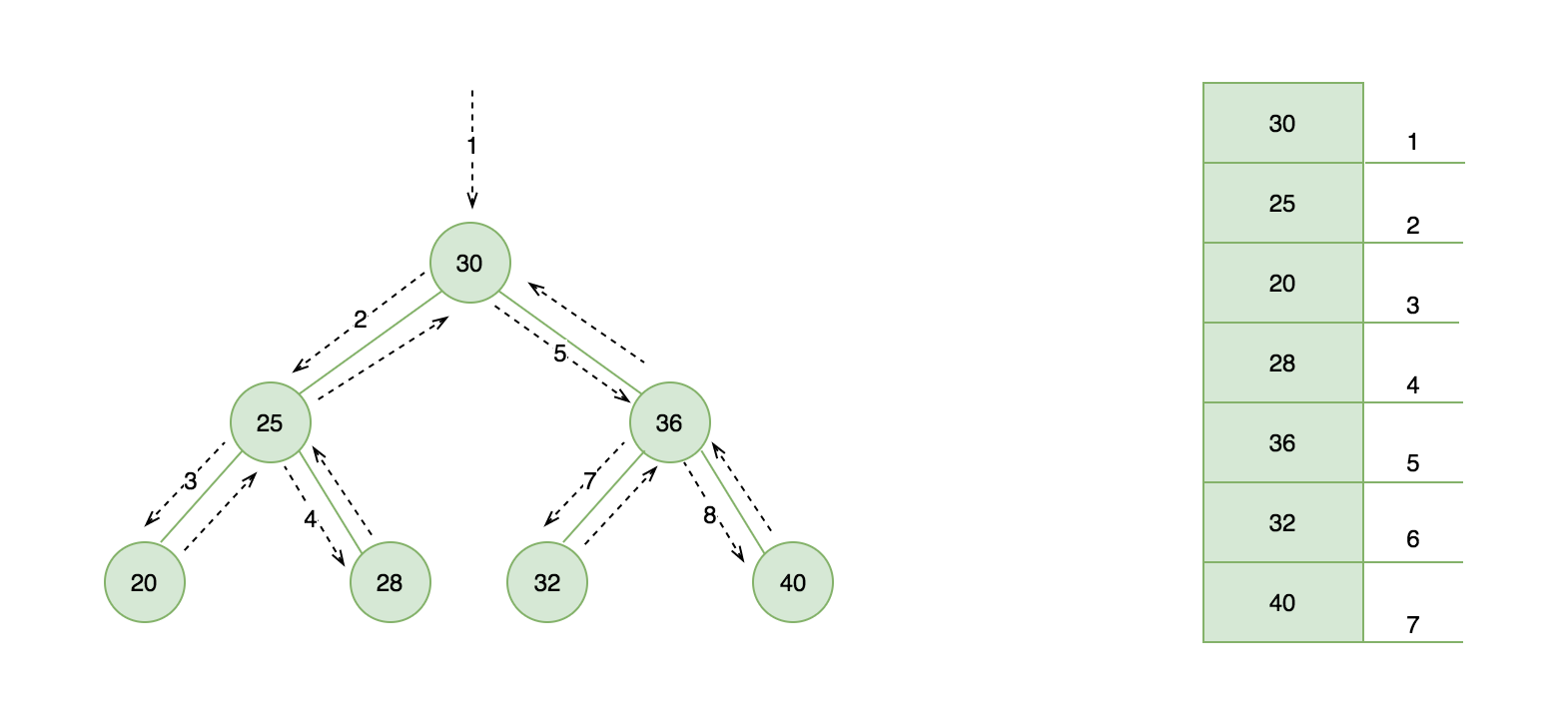### 中序遍历

```/**
* 中序遍历
* @param { Function } cb
*/
inOrderTraverse(cb) {
return this[IN_ORDER_TRAVERSE_RECUSIVE](this.root, cb);
}
```

• 行 {1} 访问左侧节点
• 行 {2} 访问节点本身
• 行 {3} 访问右侧节点
```/**
* 中序遍历递归调用
* @param { Object } node
* @param {Function } cb
*/
[IN_ORDER_TRAVERSE_RECUSIVE](node, cb) {
if (node !== null) {
this[IN_ORDER_TRAVERSE_RECUSIVE](node.left, cb); // {1} 访问左侧节点
cb(node.value); // {2} 取当前树的子节点的值（树的最底端）
this[IN_ORDER_TRAVERSE_RECUSIVE](node.right, cb); // {3} 访问右侧节点
}
}
```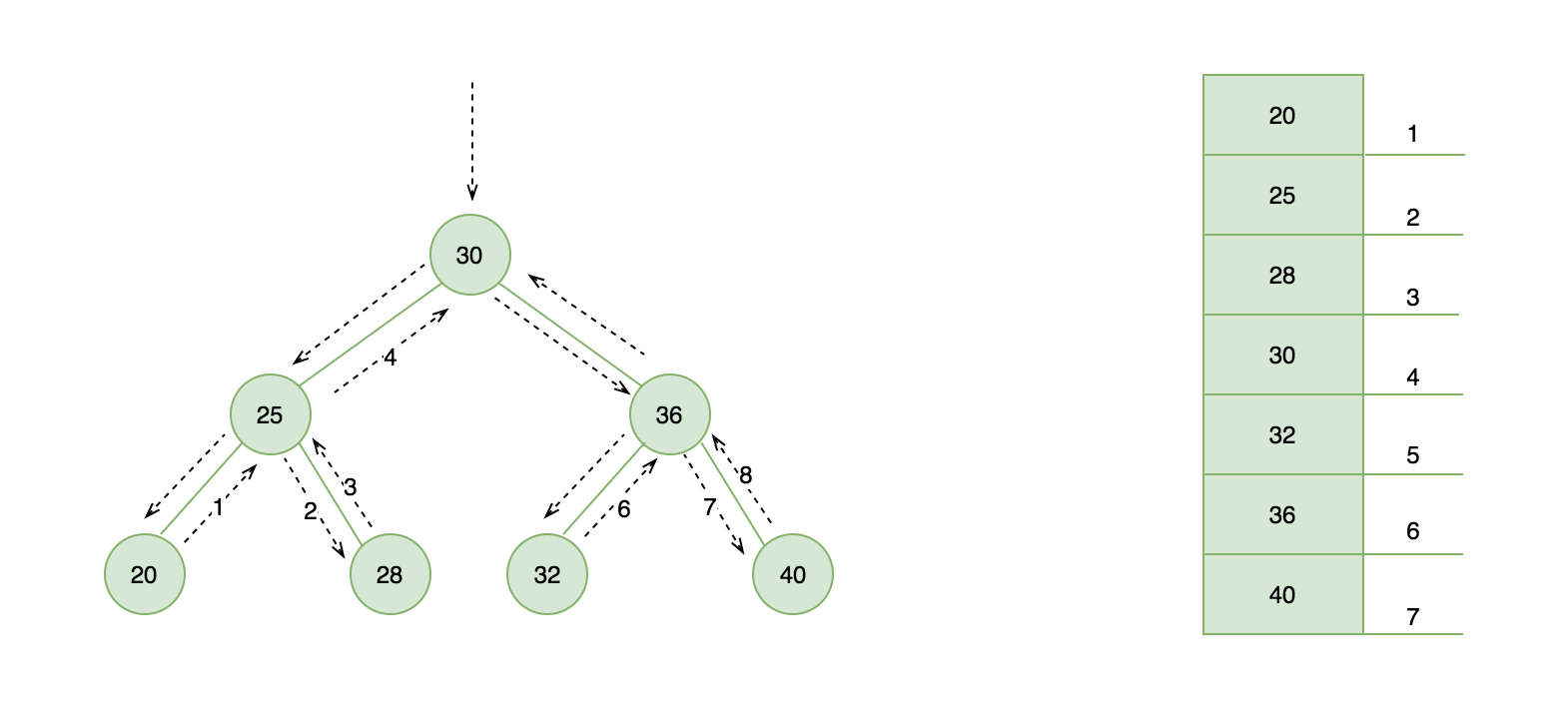### 后序遍历

```/**
* 后序遍历
* @param { Function } cb
*/
postOrderTraverse(cb) {
return this[POST_ORDER_TRAVERSE_RECUSIVE](this.root, cb);
}
```

• {1} 访问左侧节点
• {2} 访问右侧节点
• {3} 取当前节点本身
```/**
* 后序遍历递归调用
* @param {*} node
* @param {*} cb
*/
[POST_ORDER_TRAVERSE_RECUSIVE](node, cb) {
if (node !== null) {
this[POST_ORDER_TRAVERSE_RECUSIVE](node.left, cb); // {1} 访问左侧节点
this[POST_ORDER_TRAVERSE_RECUSIVE](node.right, cb); // {2} 访问右侧节点
cb(node.value); // {3} 取当前节点本身
}
}
```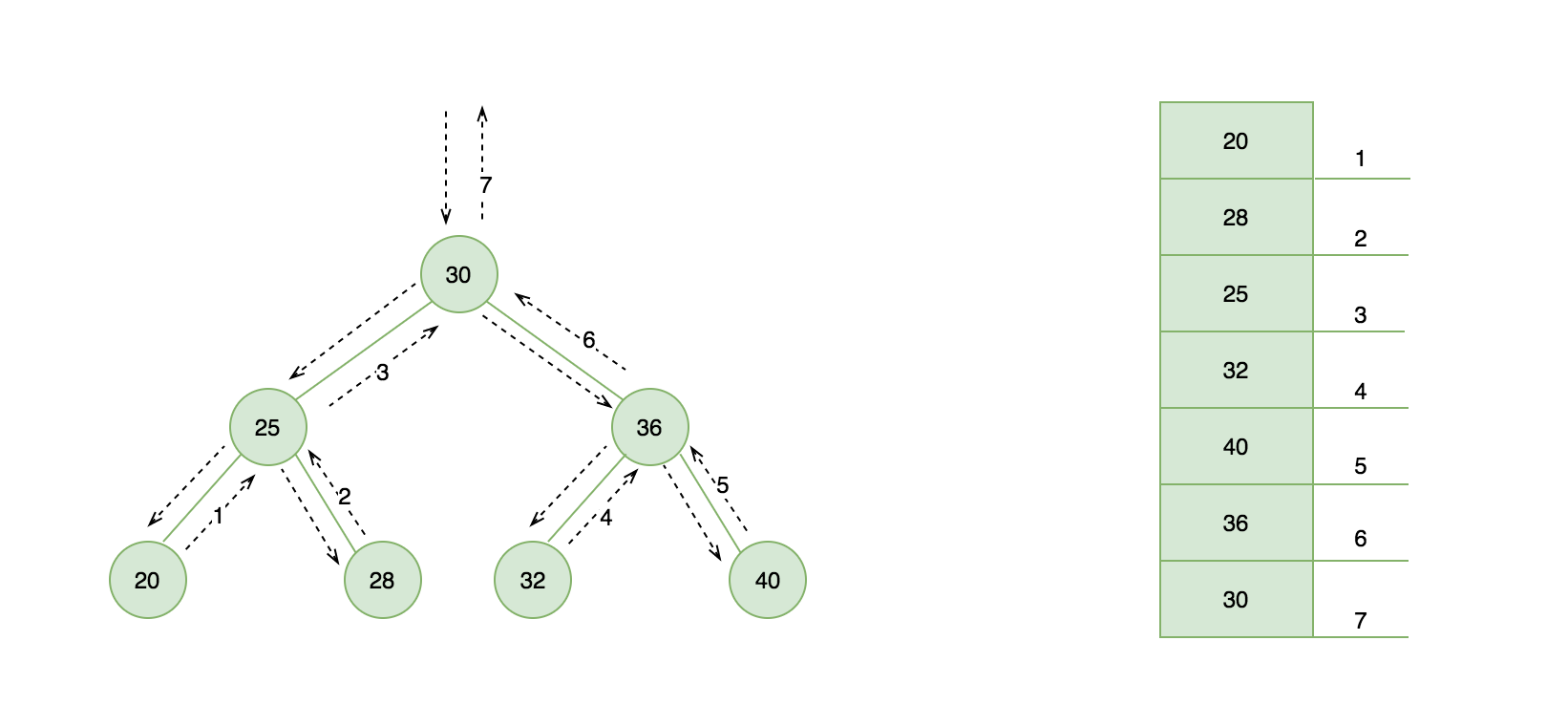## 二叉树搜索销毁

```/**
* 二叉树销毁，可以利用后续遍历特性实现
*/
destroy(){
this.root = this[DESTORY_RECUSIVE](this.root);
}
```

```/**
* 销毁二叉搜索树递归调用
* @param { Object } node
*/
[DESTORY_RECUSIVE](node) {
if (node !== null) {
this[DESTORY_RECUSIVE](node.left);
this[DESTORY_RECUSIVE](node.right);

node = null;
this.count--;
return node;
}
}
```

## 最大最小节点

### 求二叉树中最小节点值

```/**
* 求二叉树中最小节点值
* @return value
*/
minNodeValue() {
const result = this.minNode(this.root);

return result !== null ? result.value : null;
}
```

```/**
* 求最小节点
*/
minNode(node) {
if (node === null) {
return node;
}

while (node && node.left !== null) {
node = node.left;
}

return node;
}
```

### 求二叉树中最大节点

```/**
* 求二叉树中最大节点
*/
maxNodeValue() {
let node = this.root;

if (node === null) {
return node;
}

while(node && node.right !== null) {
node = node.right;
}

return node.value;
}
```

## 删除节点

```/**
* 删除节点
* 若删除节点为 n，找到删除节点的后继 s = min(n->right)
*/
removeNode(value) {
this.root = this[REMOVE_NODE_RECUSIVE](this.root, value);
}
```

• {1} 先判断节点是否为 null，如果等于 null 直接返回。
• {2} 判断要删除节点小于当前节点，往树的左侧查找
• {3} 判断要删除节点大于当前节点，往树的右侧查找
• {4} 节点已找到，另划分为四种情况
• {4.1} 当前节点即无左侧节点又无右侧节点，直接删除，返回 null
• {4.2} 若左侧节点为 null，就证明它有右侧节点，将当前节点的引用改为右侧节点的引用，返回更新之后的值
• {4.3} 若右侧节点为 null，就证明它有左侧节点，将当前节点的引用改为左侧节点的引用，返回更新之后的值
• {4.4} 若左侧节点、右侧节点都不为空情况
```/**
* 删除一个节点递归调用
* @param { Object } node
* @param { Number } value
*/
[REMOVE_NODE_RECUSIVE](node, value) {
// {1} 未查找到直接返回 null
if (node === null) {
return node;
}

// {2} 左侧节点递归删除
if (value < node.value) {
node.left = this[REMOVE_NODE_RECUSIVE](node.left, value);
return node;
}

// {3} 右侧节点递归删除
if (value > node.value) {
node.right = this[REMOVE_NODE_RECUSIVE](node.right, value);
return node;
}

// {4} value === node.value 节点找到

// {4.1} 当前节点即无左侧节点又无右侧节点，直接删除，返回 null
if (node.left === null && node.right === null) {
node = null;
this.count--;
return node;
}

// {4.2} 若左侧节点为 null，就证明它有右侧节点，将当前节点的引用改为右侧节点的引用，返回更新之后的值
if (node.left === null) {
node = node.right;
this.count--;
return node;
}

// {4.3} 若右侧节点为 null，就证明它有左侧节点，将当前节点的引用改为左侧节点的引用，返回更新之后的值
if (node.right === null) {
node = node.left;
this.count--;
return node;
}

// {4.4} 若左侧节点、右侧节点都不为空情况
// s = min(n->right)
if (node.left !== null && node.right !== null) {
// 找到最小节点，切断对象引用，复制一个新对象 s
const s = new this.CopyNode(this.minNode(node.right));
this.count++;
s.left = node.left;
s.right = this[REMOVE_NODE_RECUSIVE](node.right, s.value); // 删除最小节点
node = null;
this.count--;
return s; // 返回 s 节点替换掉 node 节点
}
}
```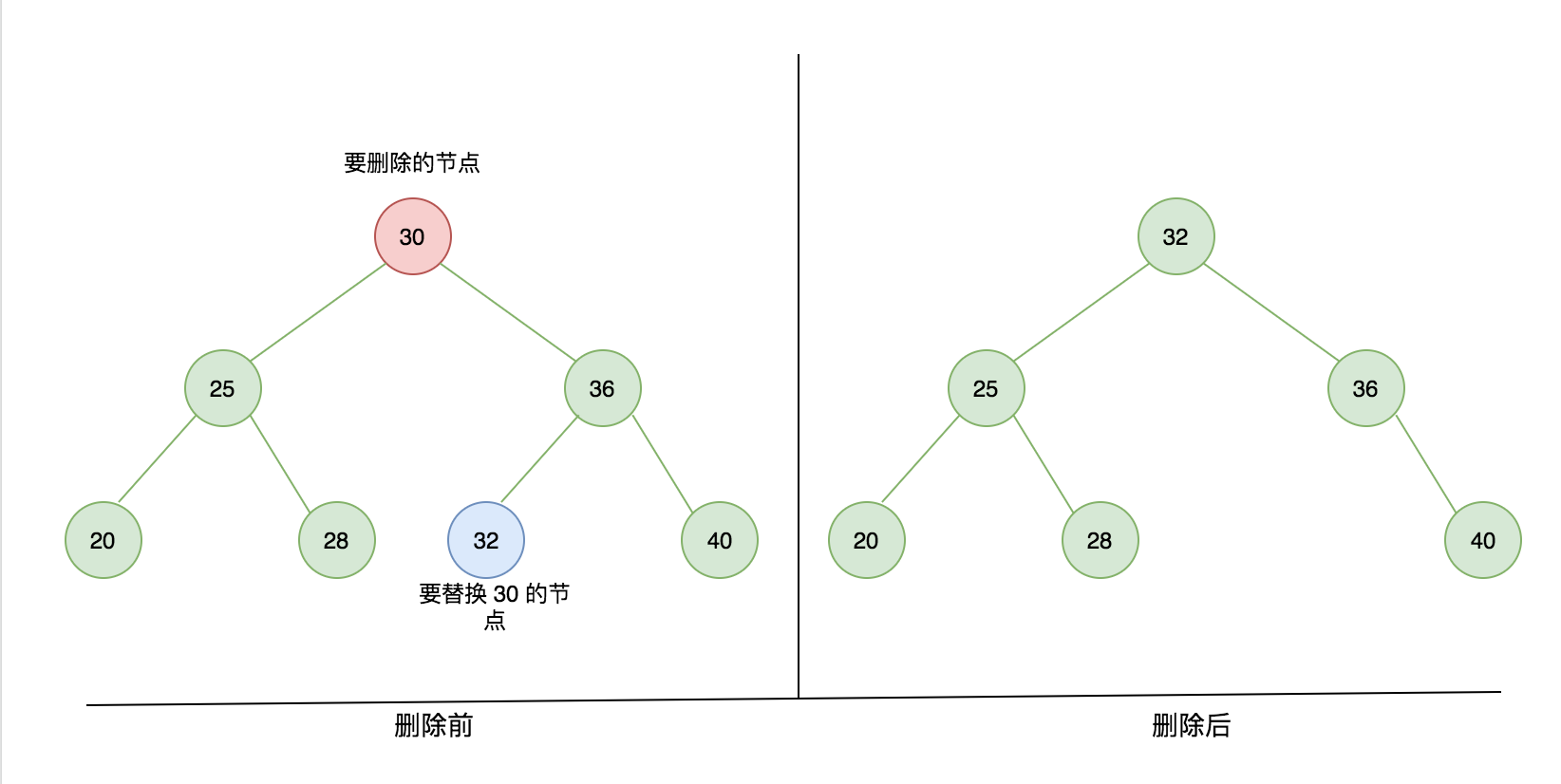## 二分搜索树局限性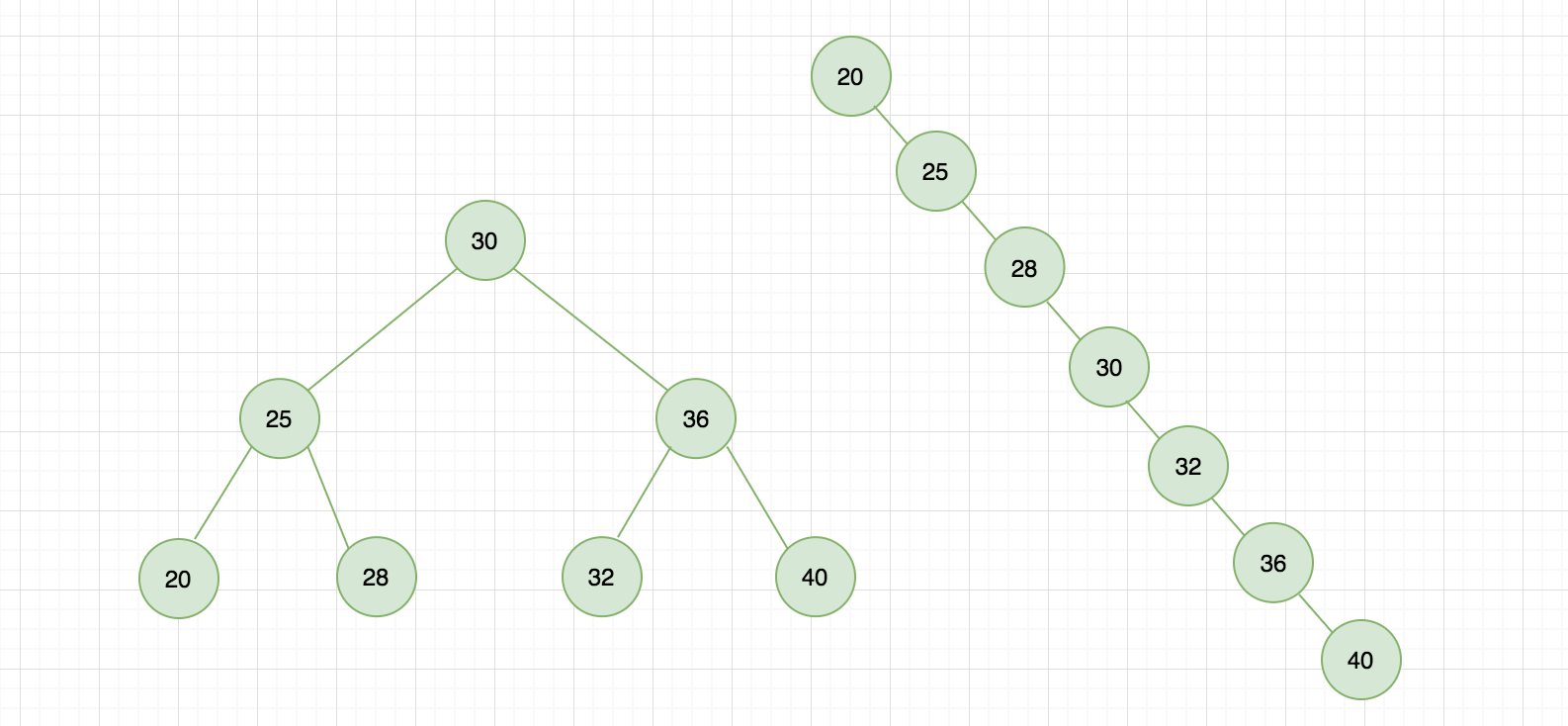`https://github.com/Q-Angelo/project-training/tree/master/algorithm/bst.js`